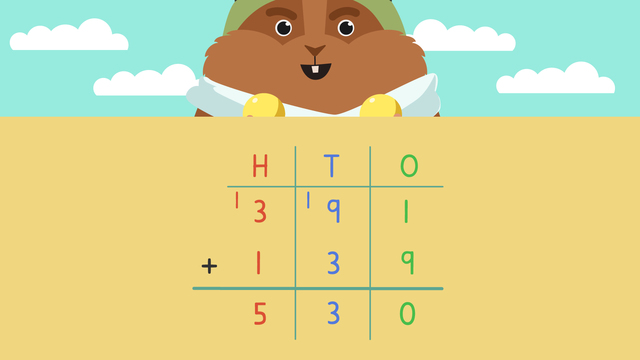# Adding 3 Digit Numbers Using Standard AlgorithmRate this video

Ø 5.0 / 2 ratings

The authorTeam Digital
Adding 3 Digit Numbers Using Standard Algorithm
CCSS.MATH.CONTENT.4.NBT.B.4

### In this Video

Mr. Squeaks is taking off on the trip of a lifetime! He is going to visit a magical place that his beloved grandpa used to tell him stories about. He’s so excited about this trip and has never traveled this far, so he wants to record every moment in his journal! We can help figure out how far away his destination, is by totaling up the distance he travels each day. In this video, we are shown how to use standard algorithm to solve 3 digit addition without regrouping and 3 digit addition with regrouping. Mr. Squeaks' travels take him to the most beautiful place he has ever seen. His grandpa would be so proud!

## Adding 3 Digit Numbers Using Standard Algorithm Exercise

Would you like to practice what you’ve just learned? Practice problems for this video Adding 3 Digit Numbers Using Standard Algorithm help you practice and recap your knowledge.
• ### What is the place value of the underlined digit?

Hints

Put your number in the columns below.

Is the underlined digit in the hundreds, tens or ones column?

Solution

If your number has 3 digits it will have a hundreds, tens and ones place.

3 0 6 Tens

3 hundreds 0 tens 6 ones

2 7 1 Hundreds

2 hundreds 7 tens 1 one

9 8 1 Ones

9 hundreds 8 tens 1 one

• ### Can you find the mistake in the solution?

Hints

Make sure the answers have been placed in the correct columns.

Since the answer from the tens column is more than 2 digits, we need to carry the tens number over to the hundreds column.

Solution

For the ones place: 3 + 5 = 8

For the tens place: 4 + 7 = 11

We need to regroup the digit in the tens place to the hundreds column. So you are left with a 1 in the tens place.

For the hundreds place: 1 + 2 + 2 = 5

500 + 10 + 8 = 518

• ### Solve the equation by placing the digits into the correct boxes.

Hints

First, make sure you have put all of the digits for the number you're adding in the correct place.

Start by adding the ones, then the tens, and lastly the hundreds place.

Don't forget if your answer in the ones or tens column is greater than 9, you need to regroup the digit in the tens of the number to the next column.

Solution

Once the digits are in the correct column:

• Add the ones column first. 4 + 3 = 7.
• Then add the tens column. 4 + 6 = 10. We need to regroup the 1 to the hundreds column. So we're left with a 0 in the tens column.
• Finally add the hundreds column. 1 + 3 + 2 = 6.

• ### Solve the equations.

Hints

For this example, looking at the answer in the tens column, the 1 in 12 needs to be regrouped to the hundreds column. Then, the hundreds column will be 1 + 7 + 6.

Are there any that need to be regrouped for these equations above?

Start adding the ones place first.

Solution

473 + 326 = 799

306 + 291 = 597

651 + 367 = 1,018

• ### What's the solution?

Hints

Add the digits in the ones column first.

3 + 5 =

Add the digits in the tens column next.

4 + 2 =

Solution

Add the digits in the ones column first 3 + 5 = 8

Then add the digits in the tens column 4 + 2 = 6

Finally add the digits in the hundreds column 2 + 1 = 3

243 + 125 = 368

• ### Solve the answers to the equations.

Hints

Put the numbers on top of each other in the place value chart. Make sure all digits are in the correct column.

Solution

Put the numbers on top of each other in the place value chart.

Add the digits in each column, starting with the ones, then tens, and lastly, hundreds.

For 362 + 244:

Add the ones: 2 + 4 = 6

Then add the tens: 6 + 4 = 10 We need to regroup the 1 to the hundreds column.

Lastly, add the hundreds: 1 + 3 + 2 = 6

362 + 244 = 606

______________________________

For the rest of the equations:

271 + 149 = 420

745 + 639 = 1384

549 + 207 = 756

365 + 256 = 621